Technical Article

# Circular Buffer: A Critical Element of Digital Signal Processors

November 13, 2017 by Dr. Steve Arar

## This article discusses circular buffering, which allows us to significantly accelerate the data transfer in a real-time system.

This article discusses circular buffering, which allows us to significantly accelerate the data transfer in a real-time system.

A digital signal processor is a specialized microprocessor for the kind of algorithms employed in digital signal processing (DSP). The main goal is to accelerate the calculations while keeping the power consumption as low as possible. In this article, we review a basic addressing capability of DSP processors, i.e. circular buffering, which allows us to significantly accelerate the data transfer in a real-time system.

Please note that since the acronym “DSP” stands for both “digital signal processing” and “digital signal processor," we will use the term “DSP processor” when referring to the hardware rather than the algorithm.

Since the finite-impulse-response (FIR) filtering is a common operation in DSP, we will continue our discussion based on examining the difference equation of an FIR filter. This simple example will show the typical properties of many DSP algorithms. After reviewing the problem of handling the incoming samples, we will discuss the circular buffering as an efficient solution to the problem.

### Performing FIR Filtering on a Real-Time Input

Assume that we have a four-tap filter with the following difference equation:

$$y(n)=b_{0}x(n)+b_{1}x(n-1)+b_{2}x(n-2)+b_{3}x(n-3)$$

##### Equation 1

where $$b_0$$, $$b_1$$, $$\dots$$, $$b_3$$ are the filter coefficients and $$x(n)$$ denotes the input samples. To calculate Equation 1, we need to store the last four samples of the input in a memory. Assume that we have a real-time system, such as a hearing aid. In this case, there is an infinite number of input samples which become available to us over time. As a result, we may take one input sample, calculate Equation 1, move the result to an output device, and then, repeat this procedure for the next input sample. Hence, this example requires the following steps for each input sample:

1. Acquire an input sample from the analog world and find its digital representation using an analog-to-digital converter (ADC).
2. Inform the system that the new sample is available.
3. Store the new sample in the memory (in the example of Equation 1, we will need this new sample to produce four output samples, so we have to store the acquired samples for some time).
4. Use the new sample and the last three samples to calculate Equation 1.
5. Move the result to an output device such as a digital-to-analog converter (DAC) or simply store it somewhere in the memory for later use.
6. Go back to step 1.

This example shows that a simple DSP algorithm involves data transfer, inequality evaluation, and a lot of math operations. For example, during steps 3 and 5, we have to transfer, respectively, the input and the result to a memory location. Step 4 involves a lot of math operations. In this step, the input samples are multiplied by their corresponding filter coefficients and the products are added together. This is generally achieved by a dedicated multiply-and-accumulate (MAC) unit which is shown in Figure 1.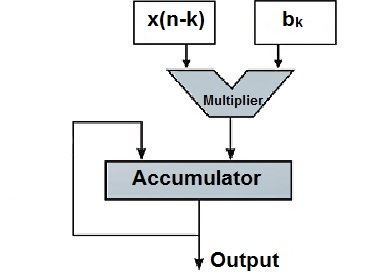##### Figure 1. The simplified model of a MAC.

Step 4 requires some inequality evaluations to keep track of the intermediate results and control the loops.

The math operations in step 4 seem to be the most time-consuming part of the algorithm and the DSP processors attempt to accelerate these calculations using various techniques. However, it is interesting to note that, without a careful design, operations such as transferring the data and controlling the loops can be time-consuming too. In the rest of this article will review a well-known technique, i.e. circular buffering, to facilitate the data transfer in a real-time system.

### Linear Buffering

The direct-form realization of Equation 1 is shown in Figure 2.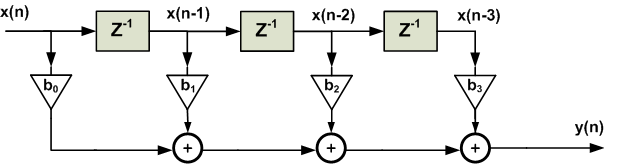##### Figure 2. The direct-form realization of a four-tap filter.

The above figure suggests that we need four memory locations to store the current sample and the three previous samples. When a new sample is acquired, we will discard the oldest sample and push the other samples upward (see Figure 3) by one memory location.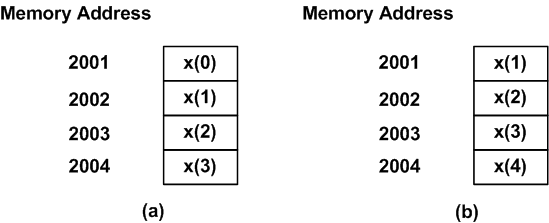##### Figure 3. A linear buffer at (a) time index n=3 and (b) n=4.

Figure 3(a) shows that, at time index $$n=3$$, the last four samples stored in the memory are $$x(3)$$, $$x(2)$$, $$x(1)$$, and $$x(0)$$. When a new sample is acquired at $$n=4$$, the last four samples, $$x(4)$$, $$x(3)$$, $$x(2)$$, and $$x(1)$$, will be stored in the memory as shown in Figure 3(b). This approach to storing the incoming samples is called the linear buffering. As we will see in a minute, it is simple but not at all efficient.

The main problem with linear buffering is the amount of the data transfer that we need to handle. Consider the above linear buffer for the four-tap FIR filter. With each new sample, we have to read three memory locations and write their contents to another location in our array. This is shown in Figure 4. We observe that the number of read and write operations are proportional to the length of the filter. That’s why the linear buffering is not an efficient method of storing the incoming samples. For example, if we use a linear buffer to implement a 256-tap filter, then, with each new sample acquired, we have to perform almost 256 read and write operations!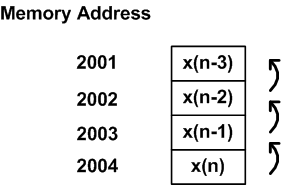### Circular Buffering

Let’s examine Figure 3 one more time. The memory in Figure 3(a) stores four input samples: $$x(0)$$, $$x(1)$$, $$x(2)$$, and $$x(3)$$. Figure 3(b) stores $$x(1)$$, $$x(2)$$,  $$x(3)$$ along with the new sample $$x(4)$$. We observe that three values, i.e. $$x(3)$$, $$x(2)$$,  $$x(1)$$,  are common between the two cases shown in Figure 3; however different memory locations are used to store these common values.

Can we use the above observation to reduce the number of the required read and write operations? For example, what’s the point of copying $$x(3)$$ from the hypothetical memory location 2004 in Figure 3(a) to the address 2003 in Figure 3(b), and then using it in the upcoming calculations? If we keep the common values where they currently reside, then we only need to store the new sample, i.e. $$x(4)$$, in the memory. When $$x(4)$$ is acquired, we no longer need $$x(0)$$, hence, we can use the memory location 2001 to store $$x(4)$$. The result is shown in Figure 5. As shown in this figure, the newest sample, $$x(4)$$, replaces the oldest sample $$x(0)$$. This technique for storing the incoming samples is called the circular buffering. In this way, with each new sample, only a single memory write operation is required.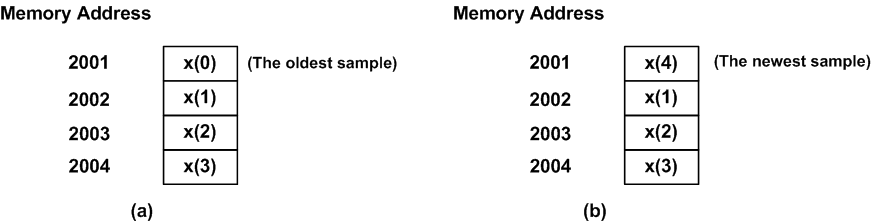##### Figure 5. A circular buffer at (a) time index n=3 and (b) n=4.

Figure 6 shows how the next four samples will be placed in the circular buffer of this example. Based on the above discussion, each new sample replaces the oldest sample. Please note the position of the newest sample in each state of Figure 6. With each new sample acquired, the position of the newest sample moves forward by one memory location from Figure 6(a) to Figure 6(c). However, when the new sample position reaches the end of the buffer in Figure 6(c), it jumps back to the beginning of the allocated memory. We can think of this as a hypothetical circular memory where the end of the buffer is connected to its beginning (Figure 7). When we reach the end the buffer, the next memory location will be the first location of the buffer. This explains the name chosen for this technique, i.e. the circular buffering.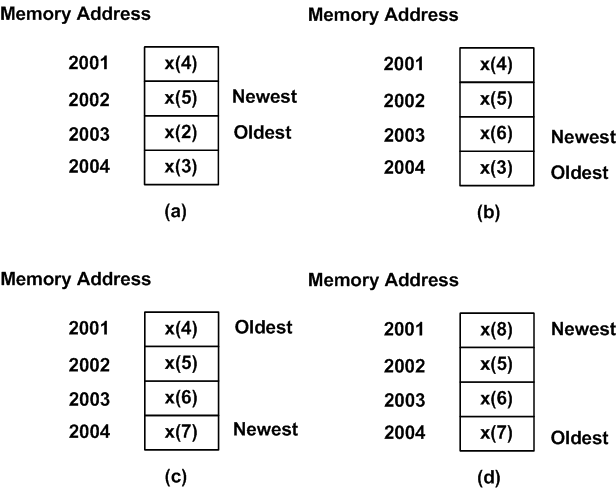##### Figure 6. The circular buffer at (a) time index n=5, (b) n=6, (c) n=7, and (d) n=8.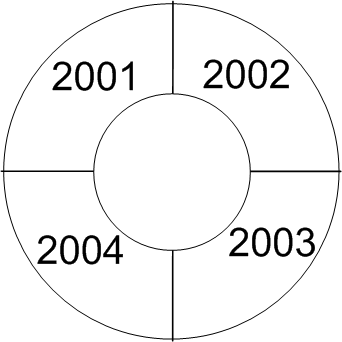### A Pointer to Interpret the Content of the Circular Buffer

With the linear buffering, each memory location corresponds to a particular time shift relative to the current sample. This doesn’t change with the time index at which we are examining the memory. For example, the memory location 2004 corresponds to the current input sample both in Figure 3(a) and (b). That’s why, in Figure 4, we can name the memory location 2004 as x(n), the memory location 2003 as x(n-1), and so on. With the circular buffering, this is not the case. For example, in Figure 5(a), the newest sample resides at memory location 2004; however, in Figure 5(b), the newest sample is at the address 2001. Hence, while the circular buffer contains all the required samples of the input to perform the FIR filtering, we have to determine the time shift associated with each memory location at a given time index. This can be easily achieved by defining a pointer to the location of the newest sample. A pointer is a variable whose value is an address. For example, to recognize the newest sample of the array in Figure 6(a), we need to set the value of the pointer to 2002. As we acquire a new sample in Figure 6(b), the pointer value will increase by one to point to the memory location 2003 and so on. Obviously, due to the circular behaviour of the buffer, the pointer must jump back to the beginning of the buffer when the pointer reaches the last memory location. Hence, in addition to a pointer to the newest sample of the array, we should also be able to recognize the first and the last location of the buffer. To this end, we can use two other pointers to store the address of the first and the last memory locations. Alternatively, we can use a pointer to store the first memory location and a variable to store the length of the circular buffer.

### A DSP Processor Versus a General Purpose Processor

When implementing a real-time system, we find a circular buffer critical whether we are using a DSP processor or a general purpose processor (GPP). However, with a GPP we may have to implement the circular buffer in software. As discussed in the previous section, with each new sample, we have to update the pointer which contains the address of the newest sample. With a circular buffer implemented in software, the programmer needs to take care of updating the buffer pointers after each read and write operation. When the pointer reaches the end of the buffer, the program must wrap the pointer back to the beginning of the buffer.

In a demanding application, we may not have the time to continuously check the pointers to see if they have reached the end of the buffer and wrap them back to the beginning of the buffer when necessary. As a result, a DSP processor uses dedicated hardware to provide some fast circular buffers. This hardware implementation automatically checks the status of the pointers and updates them accordingly. A DSP processor achieves this without using other precious resources of the system. To read more about the Analog Device’s hardware circular buffer, please see section 3.2 of this user’s manual(PDF). You can also find some coding tips to implement circular buffers in software in Appendix B of this book.

### Summary

• A digital signal processor is a specialized microprocessor for the kind of algorithms employed in DSP.
• An FIR filter involves data transfer, inequality evaluation, and a lot of math operations. Without a careful design, each of these operations can be a time-consuming process.
• The linear buffering is simple but not at all efficient.
• Circular buffering is an efficient method of storing the input data of a real-time system. Employing this technique, we need to perform only a single memory write operation for each new sample.
• With a GPP, we may have to implement the circular buffer in software. However, a DSP processor uses dedicated hardware to provide fast circular buffers.